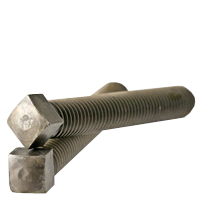1/2 DOG POINT, CASE HARDENED, PLAIN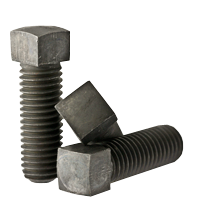CONE POINT, CASE HARDENED, PLAIN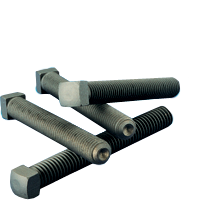CUP POINT, CASE HARDENED, PLAIN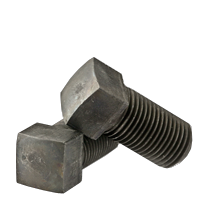CUP POINT, FINE THREAD, CASE HARDENED, PLAIN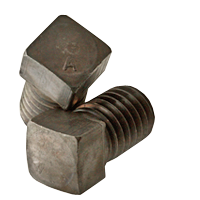CUP POINT, PLAIN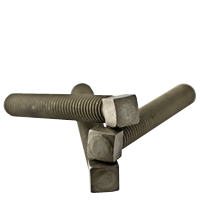FLAT POINT, CASE HARDENED, PLAIN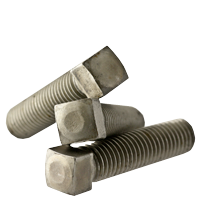OVAL POINT, CASE HARDENED PLAIN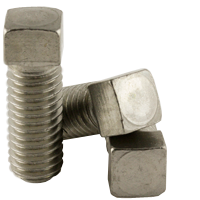STAINLESS 18 8 CUP POINT

Was: \$183.88
Now: \$165.49

Was: \$134.44
Now: \$120.99

Was: \$143.26
Now: \$128.94

Was: \$111.46
Now: \$100.32

Was: \$101.93
Now: \$91.73

Was: \$63.09
Now: \$56.78

Was: \$88.14
Now: \$79.32

Was: \$135.94
Now: \$122.35

Was: \$97.81
Now: \$88.03

Was: \$108.21
Now: \$97.39

Was: \$79.48
Now: \$71.53

Was: \$73.80
Now: \$66.42

Was: \$119.58
Now: \$107.62

Was: \$87.08
Now: \$78.37

Was: \$65.11
Now: \$58.60

Was: \$101.38
Now: \$91.24

Was: \$115.43
Now: \$103.88

Was: \$71.30
Now: \$64.17

Was: \$63.11
Now: \$56.80

Was: \$60.44
Now: \$54.39

Was: \$111.68
Now: \$100.51

Was: \$93.55
Now: \$84.20

Was: \$59.39
Now: \$53.45

Was: \$87.13
Now: \$78.41

Was: \$80.55
Now: \$72.50

Was: \$53.88
Now: \$48.49

Was: \$144.35
Now: \$129.92

Was: \$39.28
Now: \$35.35

Was: \$46.95
Now: \$42.26

Was: \$35.65
Now: \$32.09

Was: \$30.08
Now: \$27.07

Was: \$27.23
Now: \$24.50

Was: \$29.68
Now: \$26.71

Was: \$25.33
Now: \$22.79

Was: \$22.98
Now: \$20.68

Was: \$18.40
Now: \$16.56

Was: \$19.13
Now: \$17.21

Was: \$19.63
Now: \$17.66

Was: \$319.98
Now: \$287.98

Was: \$219.93
Now: \$197.93

Was: \$194.34
Now: \$174.91

Was: \$172.68
Now: \$155.41

Was: \$164.63
Now: \$148.17

Was: \$145.06
Now: \$130.56

Was: \$124.91
Now: \$112.42

Was: \$94.15
Now: \$84.74

Was: \$176.59
Now: \$158.93

Was: \$163.76
Now: \$147.39

Was: \$163.68
Now: \$147.31

Was: \$150.69
Now: \$135.62

Was: \$130.03
Now: \$117.02

Was: \$120.56
Now: \$108.51

Was: \$113.29
Now: \$101.96

Was: \$100.53
Now: \$90.47

Was: \$204.83
Now: \$184.34

Was: \$173.35
Now: \$156.02

Was: \$159.05
Now: \$143.15

Was: \$139.73
Now: \$125.75

Was: \$129.98
Now: \$116.98

Was: \$106.53
Now: \$95.87

Was: \$87.50
Now: \$78.75

Was: \$100.63
Now: \$90.56

Was: \$96.60
Now: \$86.94

Was: \$290.88
Now: \$261.79

Was: \$252.18
Now: \$226.96

Was: \$223.20
Now: \$200.88

Was: \$199.93
Now: \$179.94

Was: \$176.64
Now: \$158.98

Was: \$156.98
Now: \$141.28

Was: \$137.34
Now: \$123.60

Was: \$149.66
Now: \$134.70

Was: \$99.39
Now: \$89.45

Was: \$85.59
Now: \$77.03

Was: \$160.56
Now: \$144.51

Was: \$148.85
Now: \$133.97

Was: \$148.78
Now: \$133.90

Was: \$136.95
Now: \$123.26

Was: \$118.21
Now: \$106.39

Was: \$109.63
Now: \$98.66

Was: \$100.70
Now: \$90.63

Was: \$91.40
Now: \$82.26

Was: \$165.35
Now: \$148.82

Was: \$186.18
Now: \$167.56

Was: \$144.65
Now: \$130.19

Was: \$118.23
Now: \$106.40

Was: \$106.98
Now: \$96.28

Was: \$96.78
Now: \$87.10

Was: \$103.40
Now: \$93.06

Was: \$97.90
Now: \$88.11

Was: \$93.63
Now: \$84.26

Was: \$89.65
Now: \$80.69

Was: \$83.48
Now: \$75.13

Was: \$79.50
Now: \$71.55

Was: \$91.43
Now: \$82.28

Was: \$87.78
Now: \$79.00

Was: \$78.38
Now: \$70.54

Was: \$72.60
Now: \$65.34

Was: \$277.08
Now: \$249.37